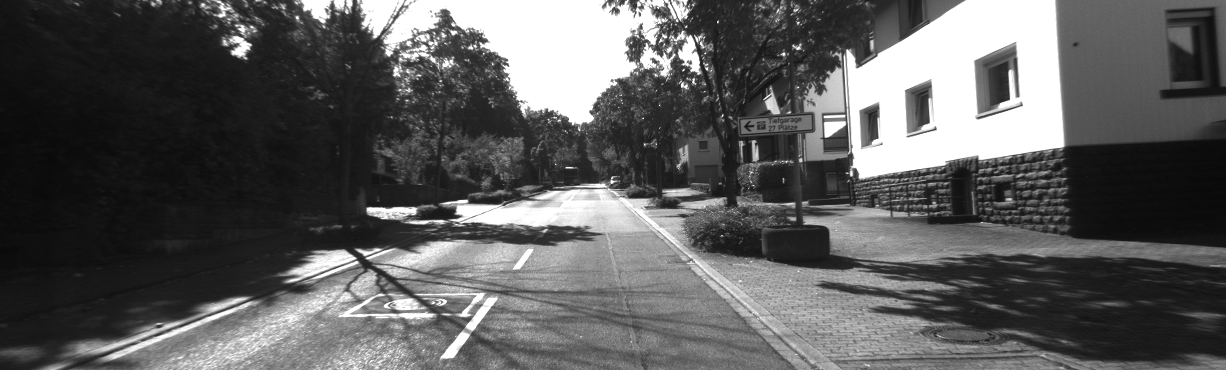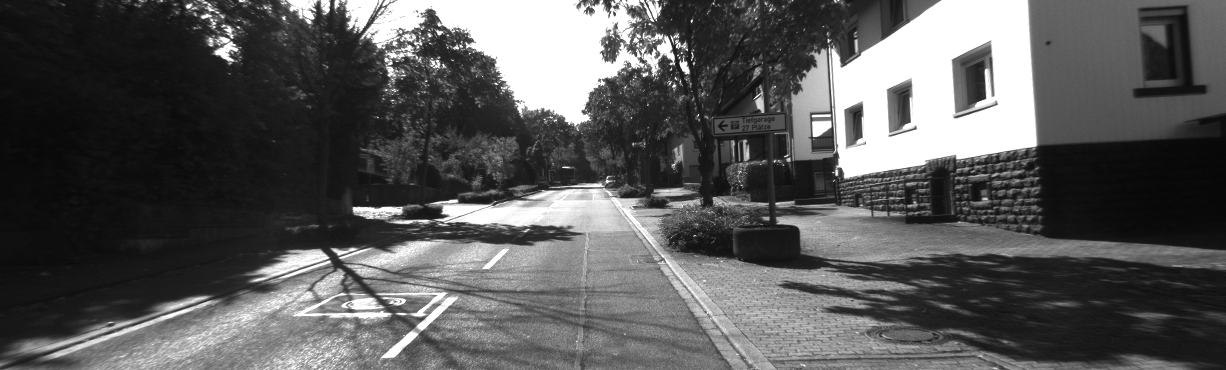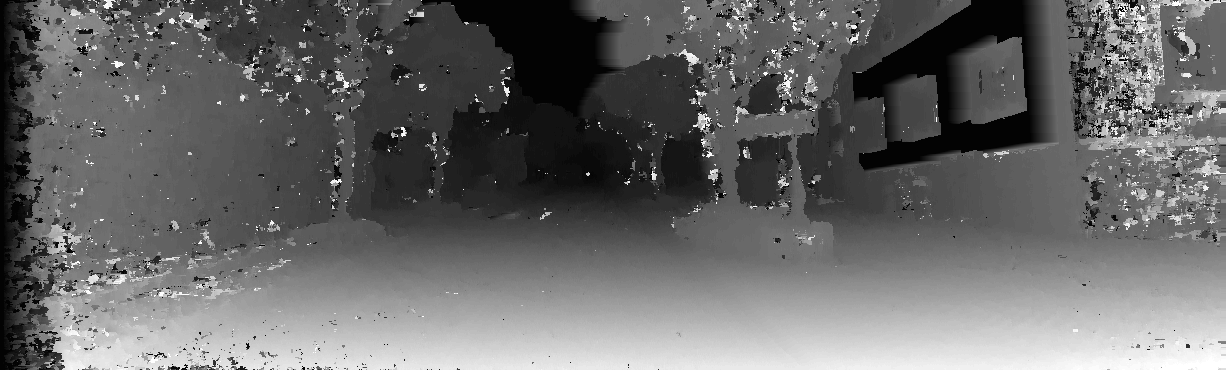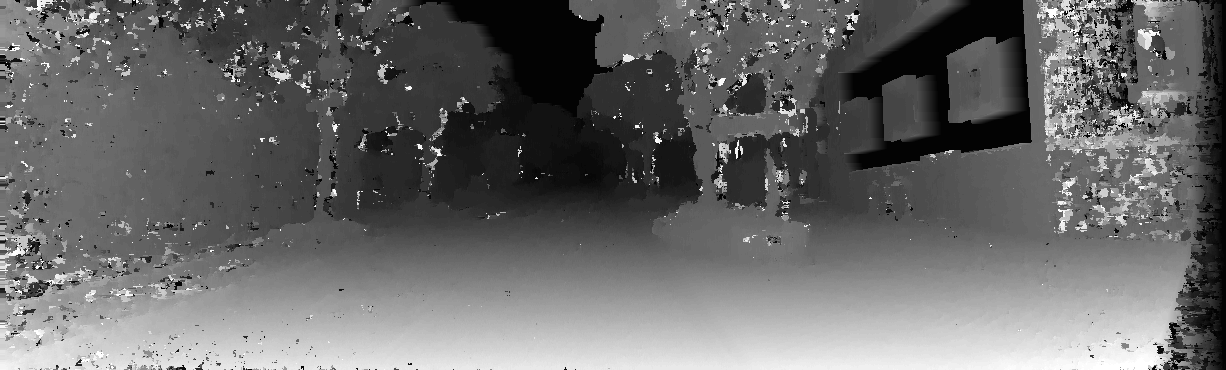# mc-cnn

### Statistics on mc-cnn

Number of watchers on Github 423
Number of open issues 32
Average time to close an issue 4 days
Main language
Open pull requests 1+
Closed pull requests 0+
Last commit about 2 years ago
Repo Created almost 4 years ago
Repo Last Updated over 1 year ago
Size 1.41 MB
Organization / Authorjzbontar
Contributors1
Page Updated
Do you use mc-cnn? Leave a review!
View open issues (32)
View mc-cnn activity
View on github
Fresh, new opensource launches 🚀🚀🚀
Trendy new open source projects in your inbox! View examples

## Subscribe to our mailing list

Evaluating mc-cnn for your project?
Commits Score (?)
Issues & PR Score (?)

# Stereo Matching by Training a Convolutional Neural Network to Compare Image Patches

The repository contains

• procedures to compute the stereo matching cost with a convolutional neural network;
• procedures to train a convolutional neural network on the stereo matching task; and
• a basic stereo method (cross-based cost aggregation, semiglobal matching, left-right consistency check, median filter, and bilateral filter);

A NVIDIA GPU with at least 6 GB of memory is required to run on the KITTI data set and 12 GB to run on the Middlebury data set. We tested the code on GTX Titan (KITTI only), K80, and GTX Titan X. The code is released under the BSD 2-Clause license. Please cite our paper if you use code from this repository in your work.

``````@article{zbontar2016stereo,
title={Stereo matching by training a convolutional neural network to compare image patches},
author={Zbontar, Jure and LeCun, Yann},
journal={Journal of Machine Learning Research},
volume={17},
pages={1--32},
year={2016}
}
``````

## Compute the Matching Cost

Install Torch, OpenCV 2.4, and png++.

Run the following commands in the same directory as this README file.

Compile the shared libraries:

``````\$ cp Makefile.proto Makefile
\$ make
``````

The command should produce two files: `libadcensus.so` and `libcv.so`.

To run the stereo algorithm on a stereo pair from the KITTI 2012 training set—

• Left input image• Right input image—download the pretrained network and call `main.lua` with the following arguments:

``````\$ wget -P net/ https://s3.amazonaws.com/mc-cnn/net_kitti_fast_-a_train_all.t7
\$ ./main.lua kitti fast -a predict -net_fname net/net_kitti_fast_-a_train_all.t7 -left samples/input/kittiL.png -right samples/input/kittiR.png -disp_max 70
Writing right.bin, 1 x 70 x 370 x 1226
Writing left.bin, 1 x 70 x 370 x 1226
Writing disp.bin, 1 x 1 x 370 x 1226
``````

The first two arguments (`kitti fast`) are used to set the default hyperparameters of the stereo method. The outputs are stored as three binary files:

• `left.bin`: The matching cost after semiglobal matching and cross-based cost aggregation with the left image treated as the reference image.• `right.bin`: Same as `left.bin`, but with the right image treated as the reference image.• `disp.bin`: The disparity map after the full stereo method.Use the `bin2png.lua` script to generate the `.png` images like the ones above:

``````\$ luajit samples/bin2png.lua
Writing left.png
Writing right.png
Writing disp.png
``````

If you wish to use the raw convolutional neural network outputs, that is, without applying cross-based cost aggregation and semiglobal matching, run the following command:

``````\$ ./main.lua kitti fast -a predict -net_fname net/net_kitti_fast_-a_train_all.t7 -left samples/input/kittiL.png -right samples/input/kittiR.png -disp_max 70 -sm_terminate cnn
Writing right.bin, 1 x 70 x 370 x 1226
Writing left.bin, 1 x 70 x 370 x 1226
Writing disp.bin, 1 x 1 x 370 x 1226
``````

The resulting disparity maps should look like this:

• `left.png`• `right.png`Note that `-disp_max 70` is used only as an example. To reproduce our results on the KITTI data sets use `-disp_max 228`.

See the predict_kitti.lua script for how you might call `main.lua` in a loop, for multiple image pairs.

### Load the Output Binary Files

You can load the binary files (if, for example, you want to apply different post-processing steps that you have written yourself) by memory mapping them. We include examples of memory mapping for some of the more popular programming languages.

• Lua

``````require 'torch'
left = torch.FloatTensor(torch.FloatStorage('../left.bin')):view(1, 70, 370, 1226)
right = torch.FloatTensor(torch.FloatStorage('../right.bin')):view(1, 70, 370, 1226)
disp = torch.FloatTensor(torch.FloatStorage('../disp.bin')):view(1, 1, 370, 1226)
``````
• Python

``````import numpy as np
left = np.memmap('../left.bin', dtype=np.float32, shape=(1, 70, 370, 1226))
right = np.memmap('../right.bin', dtype=np.float32, shape=(1, 70, 370, 1226))
disp = np.memmap('../disp.bin', dtype=np.float32, shape=(1, 1, 370, 1226))
``````
• Matlab

``````left = memmapfile('../left.bin', 'Format', 'single').Data;
left = permute(reshape(left, [1226 370 70]), [3 2 1]);
right = memmapfile('../right.bin', 'Format', 'single').Data;
right = permute(reshape(right, [1226 370 70]), [3 2 1]);
disparity = memmapfile('../disp.bin', 'Format', 'single').Data;
disparity = reshape(disparity, [1226 370])';
``````
• C

``````#include <fcntl.h>
#include <stdio.h>
#include <sys/mman.h>
#include <sys/stat.h>
#include <sys/types.h>
int main(void)
{
int fd;
float *left, *right, *disp;
fd = open("../left.bin", O_RDONLY);
left = mmap(NULL, 1 * 70 * 370 * 1226 * sizeof(float), PROT_READ, MAP_SHARED, fd, 0);
close(fd);
fd = open("../right.bin", O_RDONLY);
right = mmap(NULL, 1 * 70 * 370 * 1226 * sizeof(float), PROT_READ, MAP_SHARED, fd, 0);
close(fd);
fd = open("../disp.bin", O_RDONLY);
disp = mmap(NULL, 1 * 1 * 370 * 1226 * sizeof(float), PROT_READ, MAP_SHARED, fd, 0);
close(fd);
return 0;
}
``````

## Train

This section explains how to train the convolutional neural network on the KITTI and Middlebury data sets.

### KITTI

Download both

• the KITTI 2012 data set and unzip it into `data.kitti/unzip` (you should end up with a file `data.kitti/unzip/training/image_0/000000_10.png`) and
• the KITTI 2015 data set and unzip it into `data.kitti2015/unzip` (you should end up with a file `data.kitti2015/unzip/training/image_2/000000_10.png`).

Run the preprocessing script:

``````\$ ./preprocess_kitti.lua
dataset 2012
1
...
389
dataset 2015
1
...
400
``````

Run `main.lua` to train the network:

``````\$ ./main.lua kitti slow -a train_tr
kitti slow -a train_tr
conv(in=1, out=112, k=3)
cudnn.ReLU
conv(in=112, out=112, k=3)
cudnn.ReLU
conv(in=112, out=112, k=3)
cudnn.ReLU
conv(in=112, out=112, k=3)
cudnn.ReLU
nn.Reshape(128x224)
nn.Linear(224 -> 384)
cudnn.ReLU
nn.Linear(384 -> 384)
cudnn.ReLU
nn.Linear(384 -> 384)
cudnn.ReLU
nn.Linear(384 -> 384)
cudnn.ReLU
nn.Linear(384 -> 1)
cudnn.Sigmoid
...
``````

The network is trained on a subset of all training examples with the remaining examples used for validation; to train on all examples use

``````\$ ./main.lua kitti slow -a train_all
``````

In the previous command, the KITTI 2012 data set is used. If you wish to train on the KITTI 2015 run

``````\$ ./main.lua kitti2015 slow -a train_tr
``````

To train the fast architecture instead use

``````\$ ./main.lua kitti fast -a train_tr
``````

The network is stored in the `net/` directory.

``````\$ ls net/
...
net_kitti2012_fast_-action_train_tr.t7
...
``````

### Middlebury

Run `download_middlebury.sh` to download the training data (this can take a long time, depending on your internet connection).

``````\$ ./download_middlebury.sh
``````

The data set is downloaded into the `data.mb/unzip` directory.

Compile the MiddEval3-SDK. You should end up with the `computemask` binary in one of the directories listed in your `PATH` enviromential variable.

Install ImageMagick; the preprocessing steps requires the `convert` binary to resize the images.

Run the preprocessing script:

``````\$ mkdir data.mb.imperfect_gray
\$ ./preprocess_mb.py imperfect gray
Adirondack
Backpack
...
testH/Staircase
``````

The preprocessing is slow (it takes around 30 minutes) the first time it is run, because the images have to be resized.

Use `main.lua` to train the network:

``````\$ ./main.lua mb slow -a train_tr
``````

## Other Useful Commands

Compute the error rate on the validation set (useful for setting hyperparameters):

``````\$ ./main.lua kitti fast -a test_te -net_fname net/net_kitti_fast_-a_train_tr.t7
kitti fast -a test_te -net_fname net/net_kitti_fast_-a_train_tr.t7
0.86836290359497        0.0082842716717202
...
0.73244595527649        0.024202708004929
0.72730183601379        0.023603160822285
0.030291934952454
``````

The validation error rate of the fast architecture on the KITTI 2012 data set is 3.029%.

***

Compute the error rate on the validation set of one dataset for a network that has been trained on a different dataset.

``````\$ ./main.lua kitti fast -a test_te -net_fname net/net_mb_fast_-a_train_all.t7
kitti fast -a test_te -net_fname net/net_mb_fast_-a_train_all.t7
2.1474301815033 0.0071447750148986
...
1.4276049137115 0.024273838024622
1.4282908439636 0.01881285579564
1.408842086792  0.021741689597834
0.031564540460366
``````

The validation error rate of the fast architecture tested on KITTI 2012 but trained on Middlebury is 3.156%.

***

Prepare files for submission to the KITTI and Middlebury evaluation server.

``````\$ ./main.lua kitti fast -a submit -net_fname net/net_kitti_fast_-a_train_all.t7
kitti fast -a submit -net_fname net/net_kitti_fast_-a_train_all.t7
adding: 000038_10.png (deflated 0%)
adding: 000124_10.png (deflated 0%)
...
adding: 000021_10.png (deflated 0%)
``````

The output is stored in `out/submission.zip` and can be used to submit to the KITTI evaluation server.

***

Experiment with different network architectures:

``````\$ ./main.lua kitti slow -a train_tr -l1 2 -fm 128 -l2 3 -nh2 512
kitti slow -a train_tr -l1 2 -fm 128 -l2 3 -nh2 512
conv(in=1, out=128, k=3)
cudnn.ReLU
conv(in=128, out=128, k=3)
cudnn.ReLU
nn.Reshape(128x256)
nn.Linear(256 -> 512)
cudnn.ReLU
nn.Linear(512 -> 512)
cudnn.ReLU
nn.Linear(512 -> 512)
cudnn.ReLU
nn.Linear(512 -> 1)
cudnn.Sigmoid
...
``````

***

Measure the runtime on a particular data set:

``````\$ ./main.lua kitti fast -a time
kitti fast -a time
conv(in=1, out=64, k=3)
cudnn.ReLU
conv(in=64, out=64, k=3)
cudnn.ReLU
conv(in=64, out=64, k=3)
cudnn.ReLU
conv(in=64, out=64, k=3)
nn.Normalize2
nn.StereoJoin1
0.73469495773315
``````

It take 0.73 seconds to run the fast architecure on the KITTI 2012 data set. If you care only about the time spent in the neural network, you can terminate the stereo method early:

``````\$ ./main.lua kitti fast -a time -sm_terminate cnn
kitti fast -a time -sm_terminate cnn
conv(in=1, out=64, k=3)
cudnn.ReLU
conv(in=64, out=64, k=3)
cudnn.ReLU
conv(in=64, out=64, k=3)
cudnn.ReLU
conv(in=64, out=64, k=3)
nn.Normalize2
nn.StereoJoin1
0.31126594543457
``````
mc-cnn open issues
• almost 3 years SpatialLogSoftMax & What about Multi-GPU use?
• almost 3 years a strange error while running the code
• about 3 years Error when loading module
• about 3 years a question on the performance of Middlebury dataset, especially the textureless region
• about 3 years A question about your work
• about 3 years Compiling on Jetson TX1
mc-cnn open pull requests
• Fix predict_kitti to work with new torch
mc-cnn list of languages used